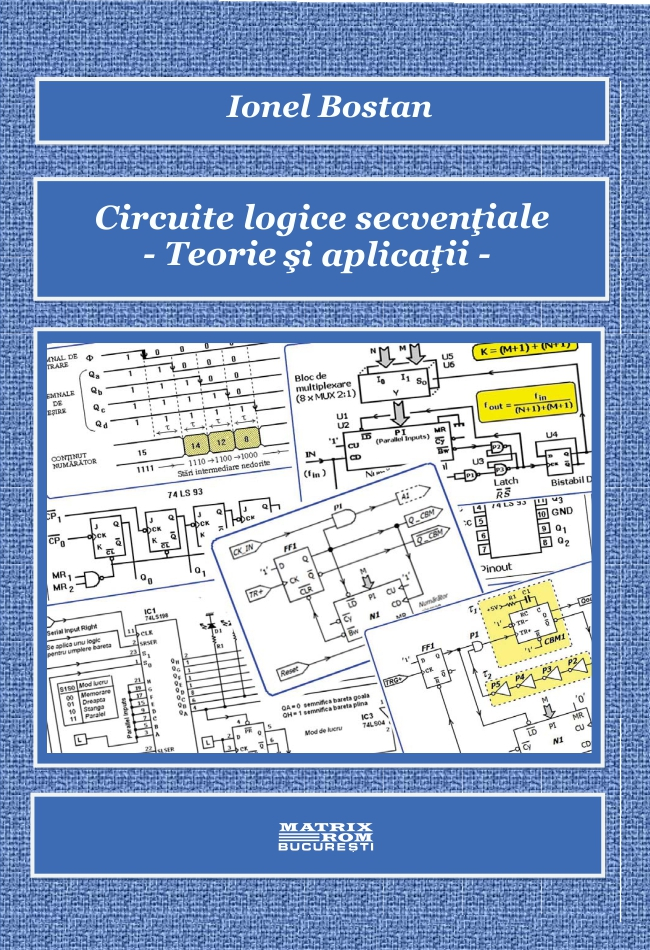Sequential logic circuits. Theory and applications | Matrix Rom
English# Sequential logic circuits. Theory and applications

Universitatea din Pitesti
Limba: Romana
Suport: Hartie
41,00 lei
The work covers the part of sequential circuits, which appears in the structure of introductory courses in the use and design of logic circuits for students majoring in electrical or computer fields. Equally, it can be useful to teachers in pre-university education as support for the discipline Basics of digital electronics.
For a better and faster understanding of sequential logic circuits, I have opted for a presentation focused predominantly on suggestive examples and solved problems, reducing general theoretical presentations to a minimum. In addition to the examples integrated in the theoretical part, at the end of each chapter, I have introduced a packet of solved problems, followed by a packet of problems proposed as homework. Overall, the work contains a lot of examples integrated in the theoretical part, to which are added over 30 problems solved at the end of the chapters, as well as 190 problems proposed as homework.